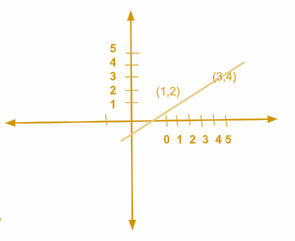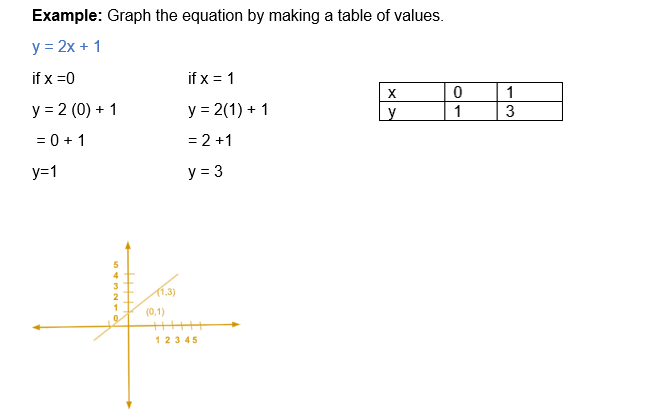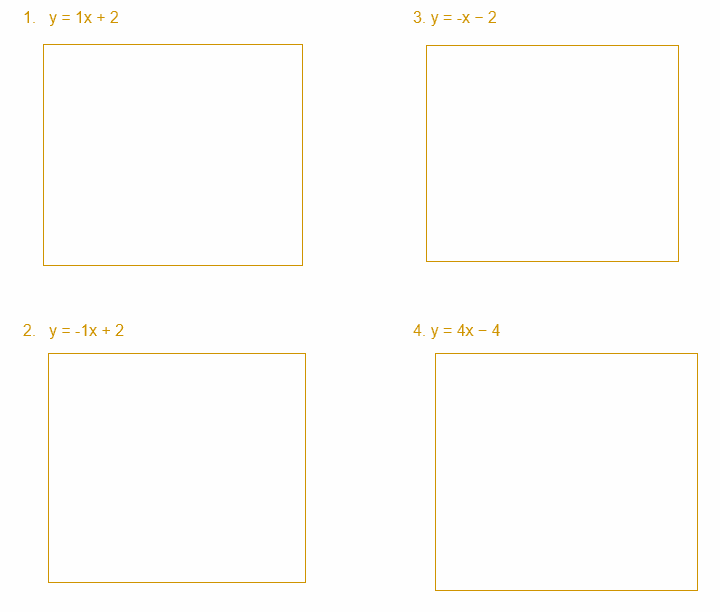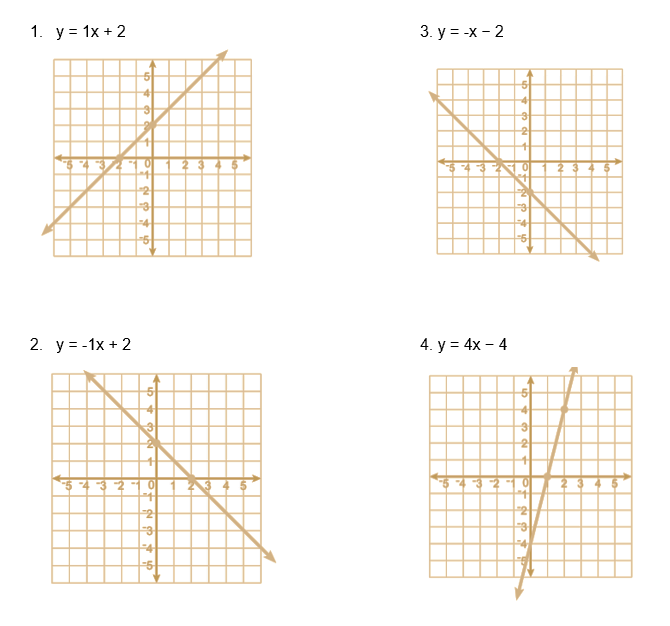# Graphing lines from Two Points Worksheet (with answer key + PDF)

Equations with one or two variables are represented on a graph through the process of graphing linear equations. An equation of degree one is referred to as a linear equation because the variable’s highest power or exponent value can never be greater than 1, in any situation. Finding the values of the variables contained in a linear equation is the process of solving it, and one way to do this is with the graphical method. This method can be used to solve linear equations with one or two variables.

## What is “Graphing lines from Two Points Worksheet”?

This worksheet will explore some of the graphing lines from two points.

## What are the graphing lines from two points?

Any linear equation, such as y=3x+2 or y=x+9, has a graph that is a line, and a line can be defined by just two points. By selecting values for x, the goal of this method is to locate two points on the line.

This worksheet will help the students to understand the topic. It also contains some information about methods and formulas.

## Instructions on how to use the “Graphing lines from Two Points Worksheet.”

Study the concept and examples given and try to solve the given exercises below.

## Conclusion

Any two points determine a line, which students should already be aware of. Finding two pairs of values and drawing a line through the points they delineate is all that is necessary to graph a linear equation. Every other point along the line will give x and y values that satisfy the equation.

If you have any inquiries or feedback, please let us know.

## Graphing lines from Two Points Worksheet (with answer key + PDF)

Graphing Linear Equation: Using Two Points

Example: Graph each linear equation that passes through the given pair of points.

(1,2) and (3,4)## Worksheet

Instructions: Graph each linear function on the coordinate plane. (Hint: you only need to plot two points to graph the line. Then you can use a ruler to draw a straight line through those two points.)